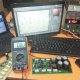Breaking News
Home / Amplifier Diagram / 2n3055 amplifier circuit diagram 200 watts.

# 2n3055 amplifier circuit diagram 200 watts.

## 2n3055 amplifier circuit diagram 200 watts.

This is a 2n3055 amplifier circuit diagram 200 watts. here used PNP and NPN transistor 2n3055 and mj2955. both are metal transistors. Complementary Silicon Power Transistors.this transistor is 15-ampere power transistors supplementary silicon 60 volts, 115 watts. here in this amplifier circuit, we are using 35-0-35 voltage and 6 amperes. 2n3055 transistor is NPN transistor and mj2955 is PNP transistor.

We know that Voltage X amperes= Watts. So, 35X6=210 Watts. So our amplifier should be 210 Watts. but it’s a mono amplifier. If we want to make a stereo amplifier then we have to use 2 circuits. one for the right channel and another for the left channel.

This is transistors.

This is circuit diagram.

To making this circuit.

We have another post for you. like as  Stk 4101   repairing amplifier,